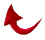74:35 This is one of the great miracles

God’s mathematical miracle of the Quran is indeed one of the great miracles.  For the past few months, I have been sharing with you my findings regarding the mathematical aspects of the Quran related to primes and their indices.  In this article, again based on this abstract mathematical approach, we will see by God’s grace that the number of H.M.’s in the seven suras (chapters) 40-46 all initialed with H.M. must be 2147 and nothing but 2147 (19x113).  But before that, I want you to appreciate the awesomeness of the Quran’s mathematical code by appreciating the fact that little is know about primes by themselves, let alone their relations to their indices.

Prime numbers have always fascinated mathematicians because it is simply impossible to tell if a number is prime or not.  Therefore, the question of the index of a prime number is a far more sophisticated and advanced question.  This is totally beyond human understanding of mathematics.  This also puts an end to the notion that the 19-based Quranic miracle could be or is probably a statistical fluke.  Now, due to God’s infinite mercy, we have solid mathematical proof that these multiples of 19 are not just any multiples of 19, but a specific multiple of 19 that God in His infinite wisdom rendered it impossible to imitate or tamper with.

Let me go back to Suras 40-46.  As we know, all these seven suras start with the initials H.M.  Sura 42 is the only sura in the Quran that has two sets of initials.  Furthermore, we know that the number of H.M.’s in each sura is not by itself divisible by 19.  We must add up the total number of H.M.’s in all seven suras which gives us 2147 = 19 x 113.  It is this coefficient that must be the prime number 113 and nothing but 113!  In the following table, we tabulate the number of H.M.’s in suras 40-46.

 Sura No. No. of H.M.’s 40 444 41 324 42 353** 43 368 44 166 45 231 46 261

Note that only 353, the number of H.M.’s in Sura 42, is a prime number.  The index of 353 is 71.  In other words, this prime number is the 71st prime number, then: 42 + 71 = 113

Furthermore, Sura 42 is the 23rd initialed sura.  Guess what? Sura 113 has 23 words and 71 letters.  So where is the prime number relation between Suras 42 and 113?  The 42nd prime number is 181 and 113th prime number is 617 and;

181 + 617 = 798

and

798 = 19 x 42

This number 798 is also the total number of initials K.H.Y.A’.S. in Sura 19.  Glory be to God.

Now, let us look at the permutation properties of the numbers which make up 2147.  These numbers are 1, 2, 4, and 7.  We can make a total of 24 four-digit numbers out of these combinations.  Only seven of these numbers are primes corresponding to the seven H.M. initialed suras, and only two are divisible by 19.  These two numbers are 2147 and 4712.  If we add these two numbers, we get;

2147 = 4712 = 6859

and

6859 = 19 x 19 x 19

Therefore, as we are witnessing here through this divine mathematical code, every single letter is by divine design, every single digit is by divine design, and the 2147 counts of H.M. initials in Suras 40-46 reported by Dr. Khalifa is the true count of the initials.  All praises are due to God, the Creator of all things.

This Quran could not possibly be authored by other that God.  It confirms all the previous messages, and provides a fully detailed scripture.  It is infallible; for it comes from the Lord of the universe.

If they say, “He fabricated it,” say, “then produce one sura like these, and invite whomever you wish, other that God, if you are truthful.” (10:37-38)Return to Table of Contents